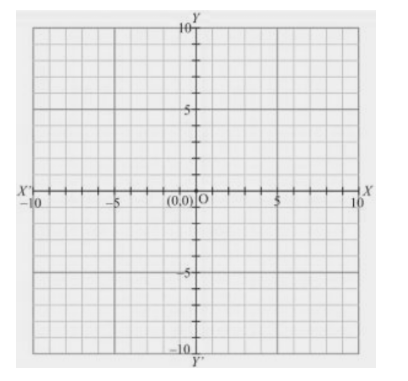# Plot the following points on the graph paper:

Question:

Plot the following points on the graph paper:

(i) (2, 5)

(ii) (4,- 3)

(iii) (- 5,- 7)

(iv) (7, - 4)

(v) (-3, 2)

(vi) (7, 0)

(vii) (- 4, 0)

(viii) (0, 7)

(ix) (0, - 4)

(x) (0, 0)

Solution:

The given points are,

A (2, 5), B (4, -3), C (-5, -7), D (7, - 4), E (- 3, 2),

F (7, 0), G (- 4, 0), H (0, 7), I (0, - 4), J (0, 0)

Let X 'OX and Y ' OY be the coordinate axes.

(i) Here for the given point the abscissa is 2 units and ordinate is 5 units. The point is in the first quadrant. So it will look like as shown in the following figure.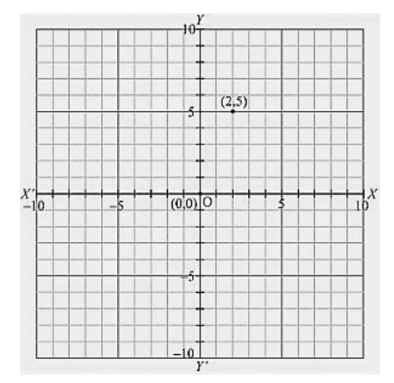(ii) Here for the given point the abscissa is 4 units and ordinate is -3 units. The point is in the fourth quadrant. So it will look like as shown in the following figure.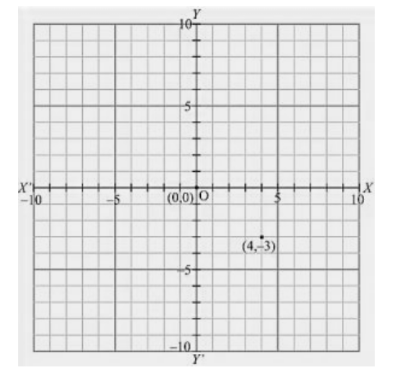(iii) Here for the given point the abscissa is -5 units and ordinate is -7 units. The point is in the third quadrant. So it will look like as shown in the following figure.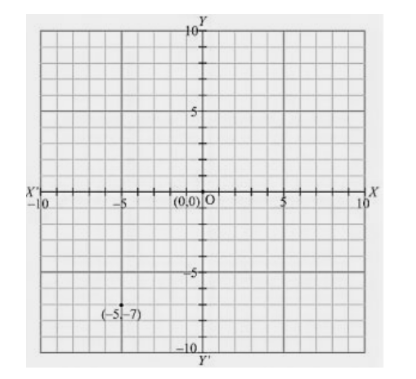(iv) Here for the given point the abscissa is 7 units and ordinate is -4 units. The point is in the fourth quadrant. So it will look like as shown in the following figure.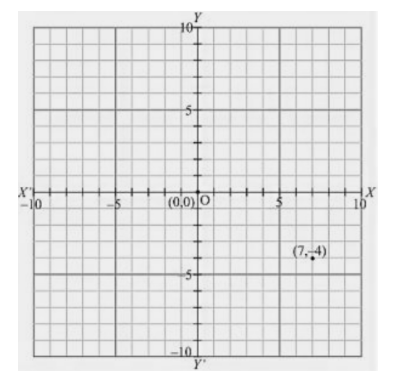(v) Here for the given point the abscissa is -3 units and ordinate is 2 units. The point is in the second quadrant. So it will look like as shown in the following figure.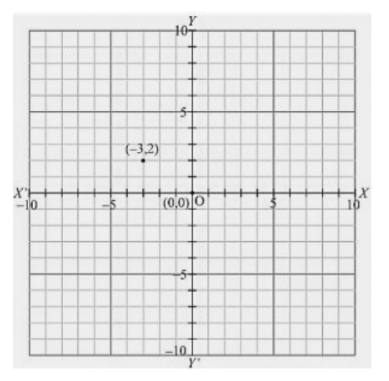(vi) Here for the given point the abscissa is 7 units and ordinate is 0 units. The point is on the x-axis. So it will look like as shown in the following figure.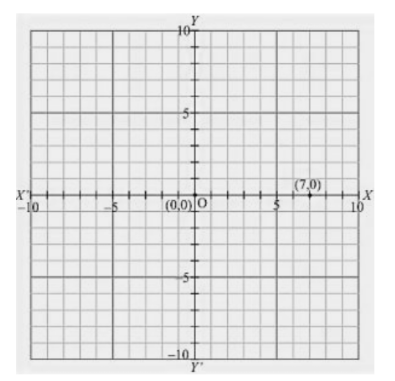(vii) Here for the given point the abscissa is - 4 units and ordinate is 0 units. The point is on the x-axis. So it will look like as shown in the following figure.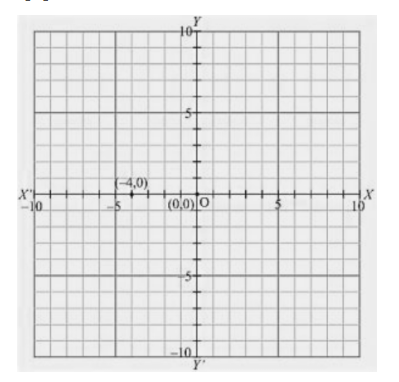(viii) Here for the given point the abscissa is 0 units and ordinate is 7 units. The point is on the y-axis. So it will look like as shown in the following figure.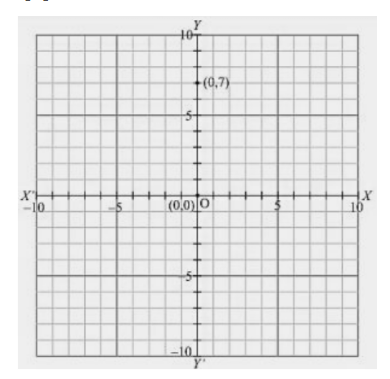(ix) Here for the given point the abscissa is 0 units and ordinate is -4 units. The point is on the y-axis. So it will look like as shown in the following figure.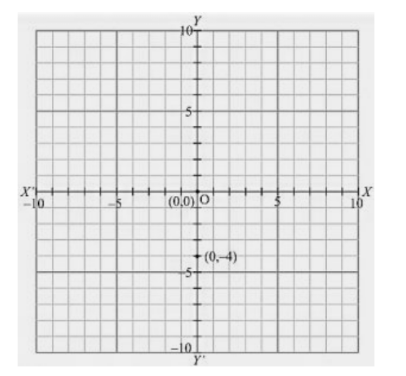(x) Here for the given point the abscissa is 0 units and ordinate is 0 units. The point is basically intersection of the coordinate axis. So it will look like as shown in the following figure